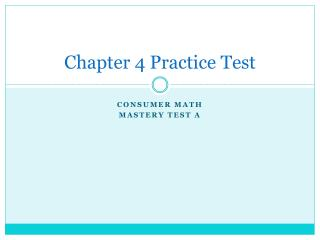DownloadDownload PresentationChapter 4 Practice Test

Chapter 4 Practice Test

Download PresentationChapter 4 Practice Test

- - - - - - - - - - - - - - - - - - - - - - - - - - - E N D - - - - - - - - - - - - - - - - - - - - - - - - - - -
Presentation Transcript

1. Chapter 4 Practice Test Consumer Math Mastery test a

2. Chapter 4 Practice Test 1. Eric’s income is \$766 every two weeks. Use the renter’s rule to compute the most Eric should spend for rent each month. \$766 ÷ 2 = \$383 * Renters Rule: The renter’s rule says you should spend no more than one week’s income for a month’s rent.

3. Chapter 4 Practice Test 2. If Eric pays \$375 for rent each month, what is his total rent paid in one year? \$375 x 12 = \$4500

4. Chapter 4 Practice Test 3. Ms. Burke earns \$32,000 per year and plans to buy a house. Use the banker’s rule to determine her maximum allowable mortgage. \$32,000 x 2.5 = \$80,000 * Banker’s Rule: The banker’s rule says that you may borrow up to 2.5 times your annual income to buy a home.

5. Chapter 4 Practice Test 4. Leo wants to buy a house for \$128,000 with a 20% down payment. How much will his down payment be? \$128,000 x .20 = \$25,600 5. What is the amount of the mortgage for Leo’s house? \$128,000 - \$25,600 = \$102,400

6. Chapter 4 Practice Test 6. What is the monthly payment on an 8% 30-year mortgage for \$68,000? \$7.34 x 68 = \$499.12 7. Compute the total amount repaid on a mortgage for \$70,000 at 9% for 35 years. \$7.84 x 70 = \$548.80 \$548.80 x 420 = \$230,496

7. Chapter 4 Practice Test 7614 8322 10. Compare the two electric meter readings above. How many units of electricity were used during the month of April? 8322 – 7614 = 708

8. Chapter 4 Practice Test 11. The natural gas rate is \$0.72 per unit. A customer uses 158 units. How much is the bill? \$0.72 x 158 = \$113.76 12. The monthly flat rate for Tim’s telephone is \$19.95. He is charged \$1.85 for a long distance call and \$1.76 for taxes. What is the total bill? \$19.95 + \$1.85 + \$1.76 = \$23.56

9. Chapter 4 Practice Test 13. Find Jessica’s utility expenses. The following amounts are due: Telephone, \$18.56 Water, \$2.73 Electricity, \$39.92 Gas, \$37.84 \$18.56 + \$2.72 + \$39.92 + \$37.84 = \$99.04

10. Chapter 4 Practice Test 14. A wood frame house in Area A; its value is \$56,800. \$56,800 x 0.0045 = \$255.60 15. A brick house in Area B; its value is \$98,000. \$98,000 x 0.0041 = \$401.80